## The Bottleneck Problem

• Attention provides a solution to the bottleneck problem, but what is the bottleneck problem?
• The bottleneck problem is that The context vector turned out to be a bottleneck for these types of models. It made it challenging for the models to deal with long sentences.
• Attention allows the model to focus on the relevant parts of the input sequence as needed.

## Attention: Under the hood

• As we stated, attention allows the model to focus on the relevant parts of the input sequence as needed. -The encoder passes a lot more data to the decoder. Instead of passing the last hidden state of the encoding stage, the encoder passes all the hidden states to the decoder:
• An attention decoder does an extra step before producing its output. In order to focus on the parts of the input that are relevant to this decoding time step, the decoder does the following
• Multiply each hidden state by its softmax score, thus amplifying hidden states with high scores, and drowning out hidden states with low scores
• Self attention is in Encoder
• Cross attention is in Decoder
• On each step of the decoder use direct connection to the encoder to focus on a particular part

## Seq2Seq with Attention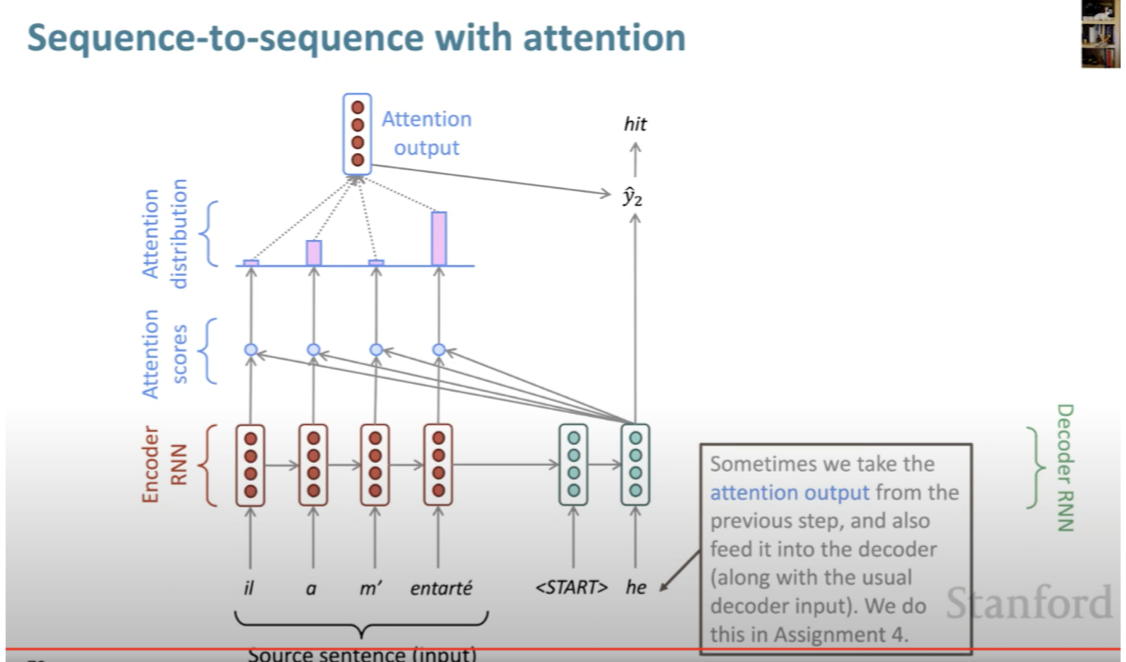• Instead of source and target sentences, we also have 2 sequences: passage and question(lengths are imbalance)
• We need to model which words in the passage are most relevant to the question (and which question words)
• Attention is the key ingredient here, similar to which words in source sentences are most relevant to the current target word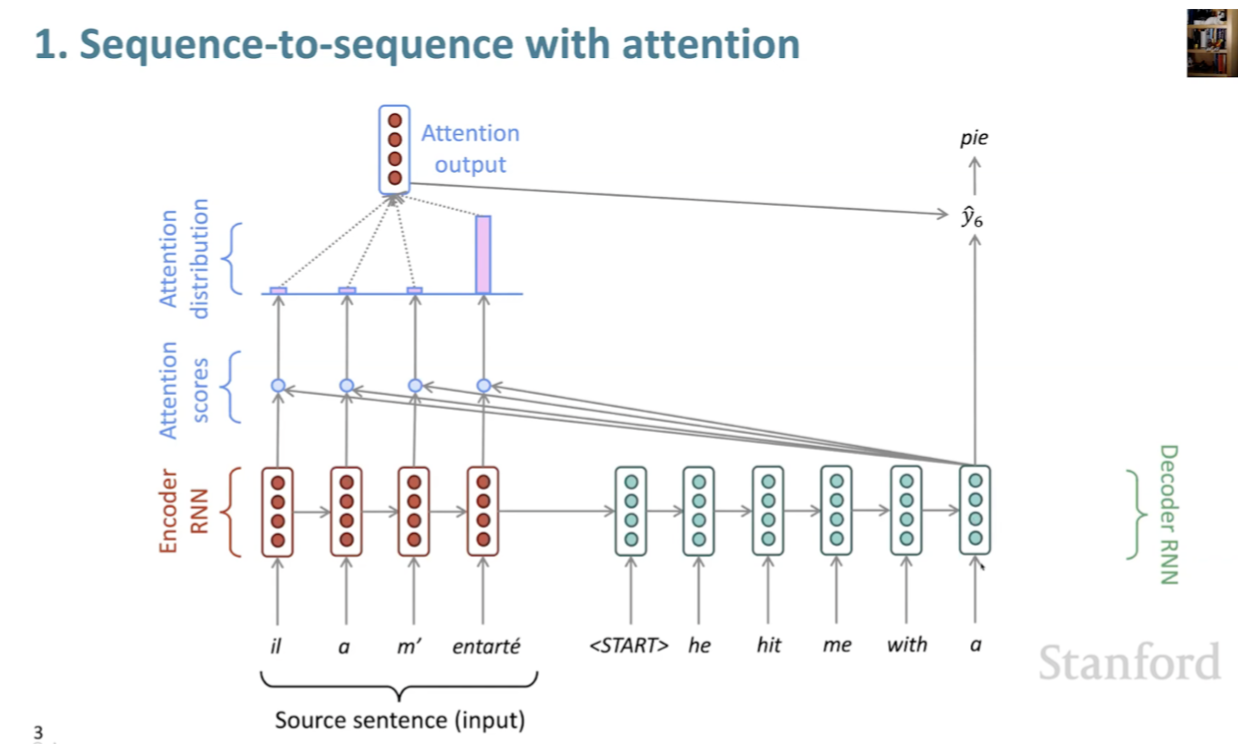## Self Attention: Under the hood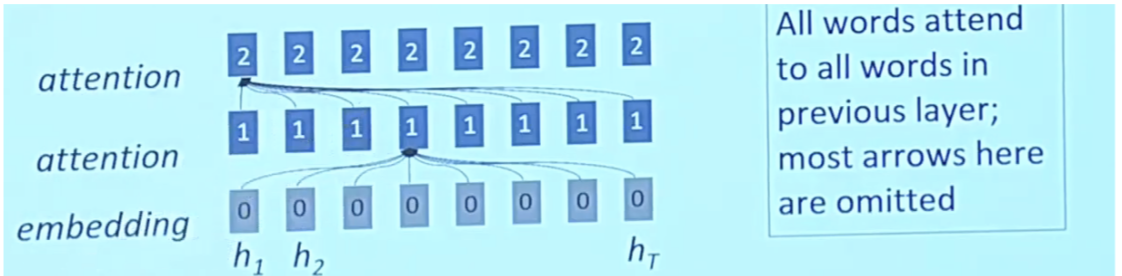• First step is to create 3 vectors from each of the encoders input vectors (embeddings of each word)
• Key, query, value vectors
• These vectors are created by multiplying the embedding by 3 matrices that we trained during the training process
• K, V, Q dimension is 64 while embedding and encoder input/output vectors have dimension of 512
• Lets look at the image below by Jay Alammar: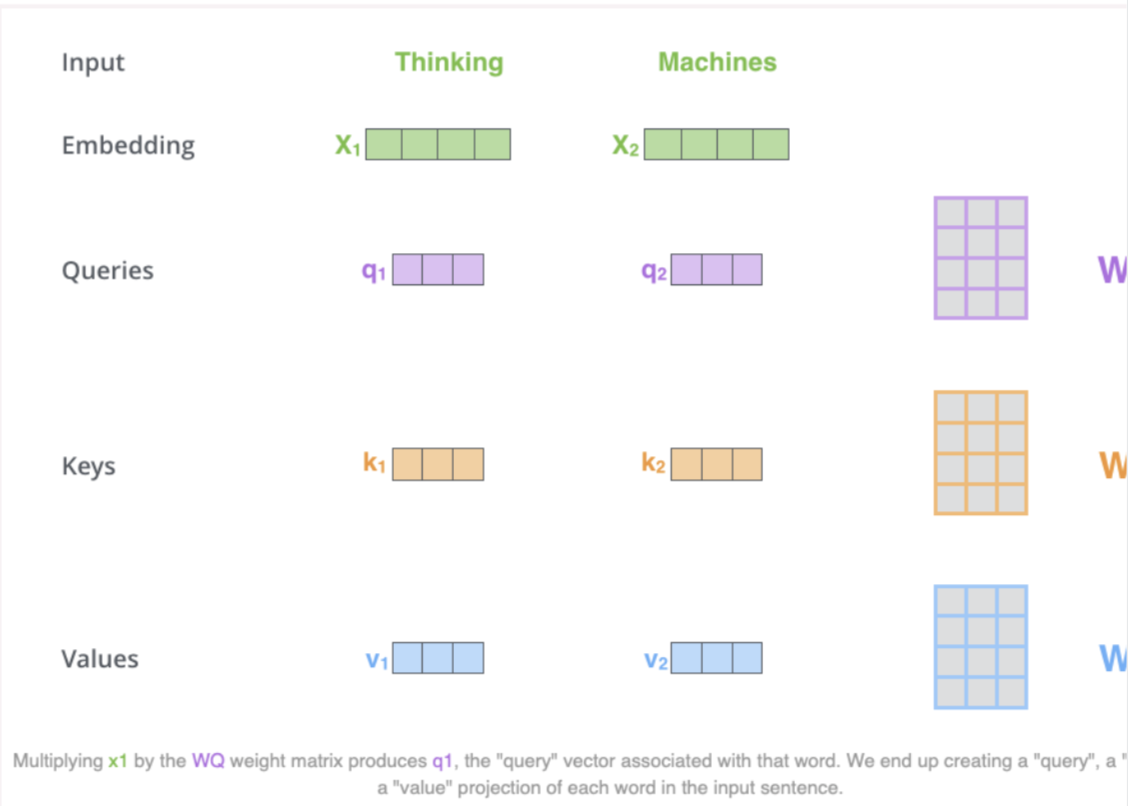• What are query, key and value vectors?
• They are abstractions that are useful for calculating and thinking about attention
• Second Step in calculating self attention is to calculate a score
• Say we’re calculating the self-attention for the first word in this example, “Thinking”. We need to score each word of the input sentence against this word.
• The score determines how much focus to place on other parts of the input sentence as we encode a word at a certain position.
• The score is calculated by taking the dot product of the query vector with the key vector of the respective word we’re scoring. So if we’re processing the self-attention for the word in position #1, the first score would be the dot product of $$q1$$ and $$k1$$.
• The second score would be the dot product of $$q1$$ and $$k2$$.
• Third and fourth steps are to divide the scores by 8 (the square root of the dimension of the key vectors used in the paper - 64) then pass the result through a softmax to normalize the scores so theyre all positive and add up to 1
• The fifth step is to multiply each value vector by the softmax score (in preparation to sum them up). The intuition here is to keep intact the values of the word(s) we want to focus on, and drown-out irrelevant words (by multiplying them by tiny numbers like 0.001, for example).
• The sixth step is to sum up the weighted value vectors. This produces the output of the self-attention layer at this position (for the first word).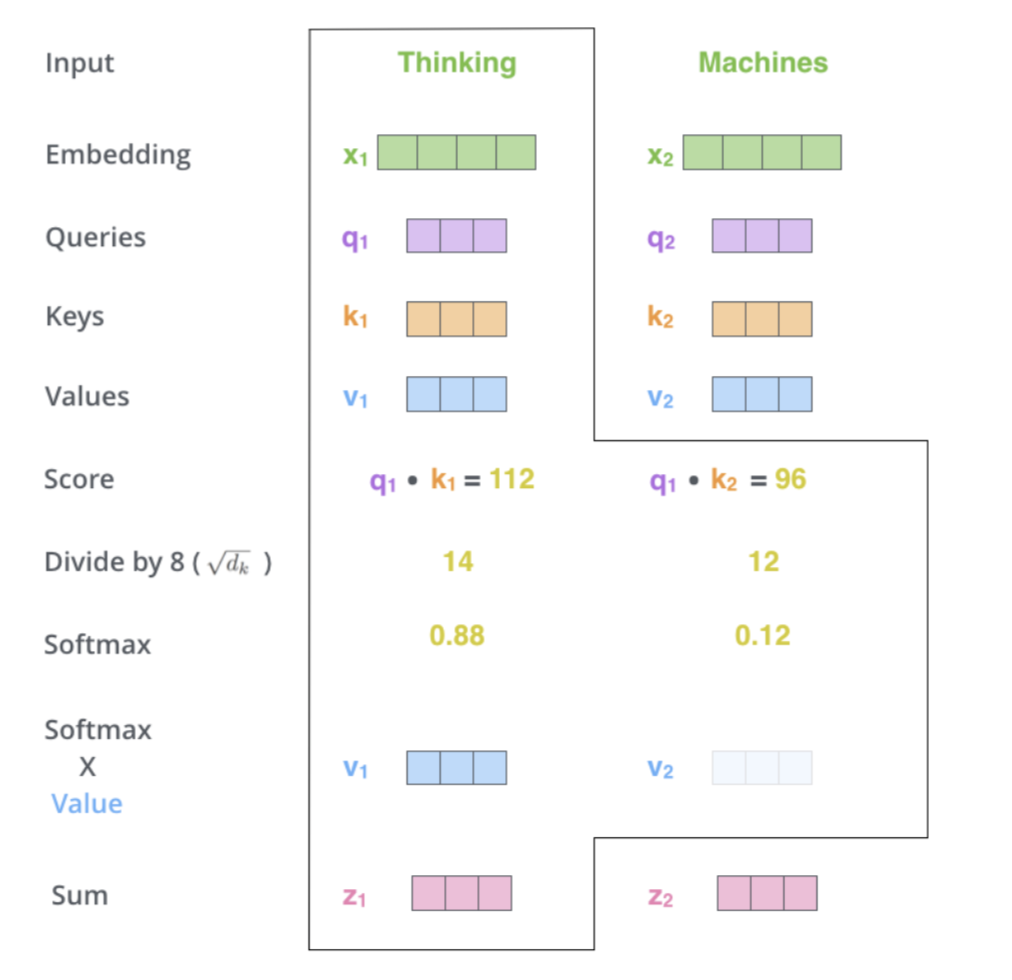• Attention operates on queries, keyes and value (all vectors)
• All $$q$$, $$k$$, and $$v$$ come form the same source, same sentence
• RNN = fully connected layer, you have your weights that you are learning slowly over the course of the network
• In attention, interaction between key and query vectors which are depend on actual content, are allowed to vary by time, and so strengths of attention weights can change as a function of the input
• Parametrization is different, instead of learning independent connection weight, instead you’re allowed to parameterize the attention as dot product functions between vectors of representations
• Throw out LSTMs and use self attention instead
• Key query value are actually just the same vector
• Self attention is a function on $$k$$, $$q$$, $$v$$
• Self attention doesn’t know the order of its inputs
• Sequence order is the first attention problem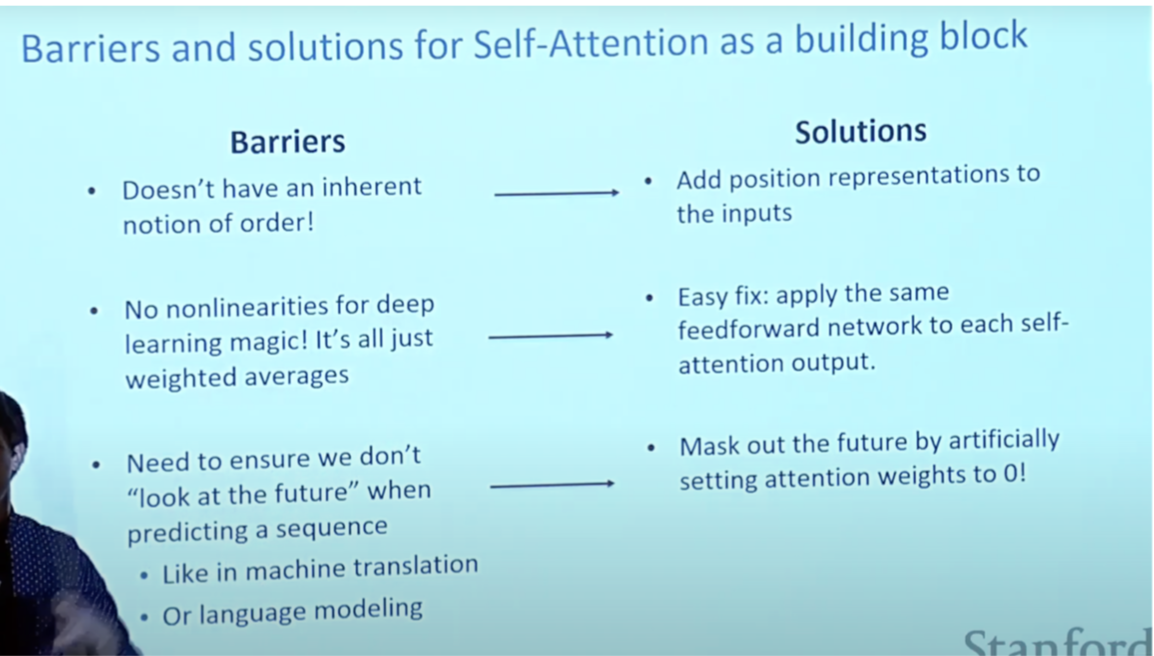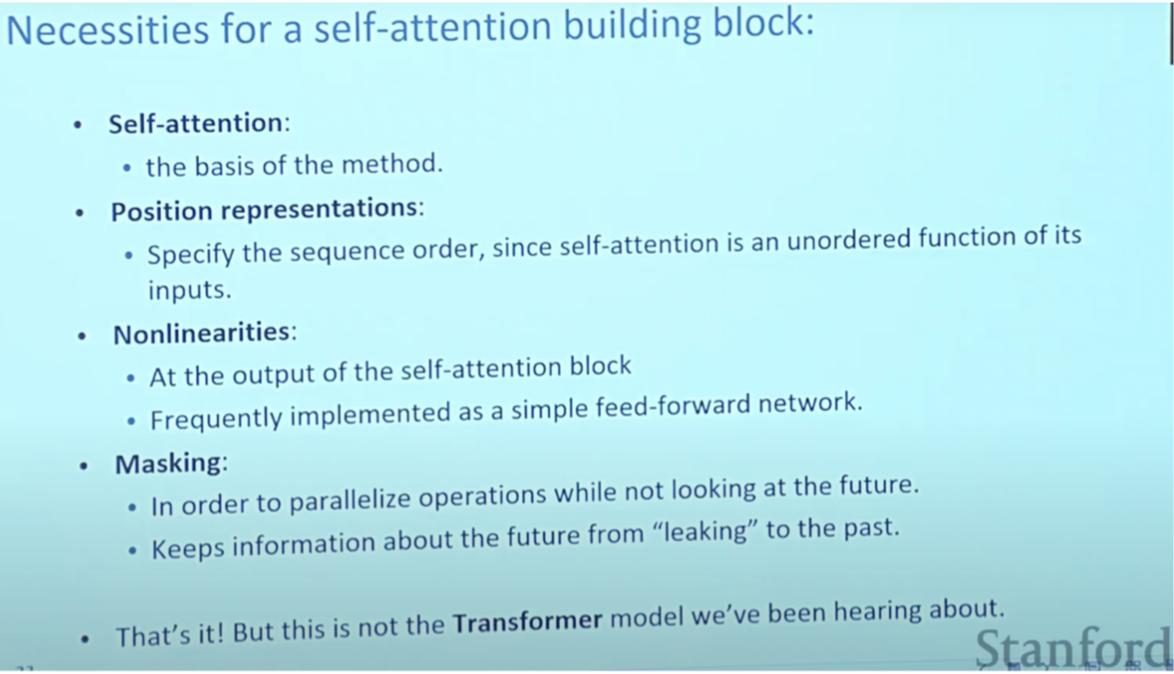• Expands the models ability to focus on different positions.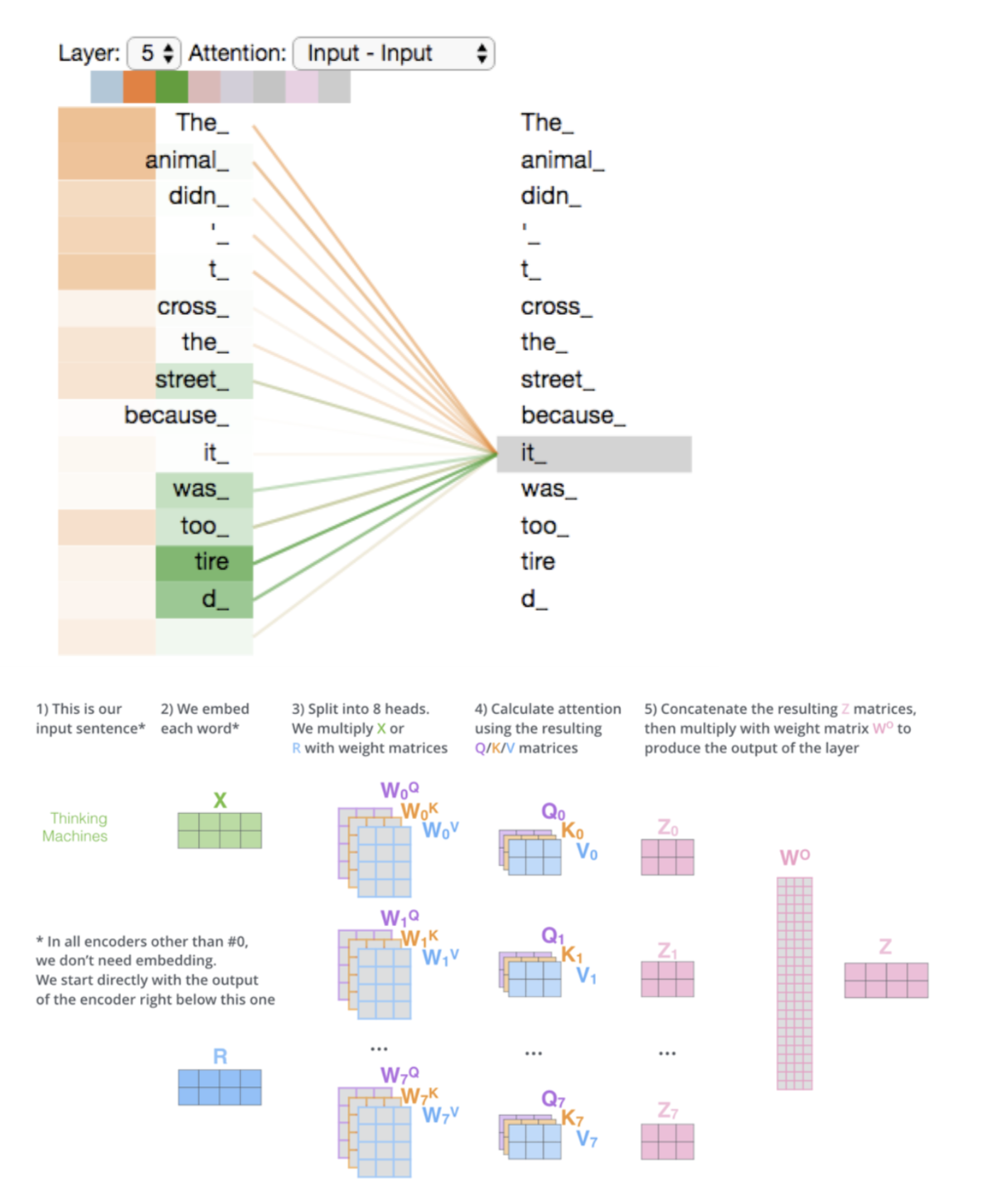• The encoder starts by processing the input sequence. The output of the top encoder is then transformed into a set of attention vectors K and V. These are to be used by each decoder in its “encoder-decoder attention” layer which helps the decoder focus on appropriate places in the input sequence
• In the decoder, the self-attention layer is only allowed to attend to earlier positions in the output sequence. This is done by masking future positions (setting them to -inf) before the softmax step in the self-attention calculation.
• The “Encoder-Decoder Attention” layer works just like multiheaded self-attention, except it creates its Queries matrix from the layer below it, and takes the Keys and Values matrix from the output of the encoder stack.
• The decoder stack outputs a vector of floats. How do we turn that into a word? That’s the job of the final Linear layer which is followed by a Softmax Layer.
• The softmax layer then turns those scores into probabilities (all positive, all add up to 1.0). The cell with the highest probability is chosen, and the word associated with it is produced as the output for this time step.
• Let’s look at an example :
• The animal didn’t cross the street because it was too tired”
• What does “it” in this sentence refer to? Is it referring to the street or to the animal? It’s a simple question to a human, but not as simple to an algorithm.
• When the model is processing the word “it”, self-attention allows it to associate “it” with “animal”.
• Self-attention is the method the Transformer uses to bake the “understanding” of other relevant words into the one we’re currently processing.
• Both CNNs and RNNs have been crucial in sequence transduction applications involving the encoder-decoder architecture. Attention-based mechanisms, as described above, have further boosted the capabilities of these models.
• However, one of the bottlenecks suffered by these architectures is the sequential processing at the encoding step. To address this, Vaswani et al. (2017) proposed the Transformer which dispensed the recurrence and convolutions involved in the encoding step entirely and based models only on attention mechanisms to capture the global relations between input and output.
• Significantly improves NMT performance
• Provides more human like model of the MT process
• Solves the bottleneck problem, allows decoder to look directly at source, bypass bottleneck
• Provides some interpretability
• Helps with vanishing gradient problem
• Always involves computing the attention scores
• Taking softmax to get attention distribution
• Using attention distribution to take weighted sum of values and thus obtaining the attention output
• Several attention variants:
• Basic dot product
• Multiplicative attention
• Reduced rank multiplicative attention
• Attention is a general Deep learning technique, can use it in many architectures not just seq2seq
• Given a set of vector values and a vector query, attention is a technique to compute a weighted sum of the values, dependent on the query

## Citation

If you found our work useful, please cite it as:

@article{Chadha2021Distilled,
title   = {Attention},
author  = {Jain, Vinija and Chadha, Aman},
journal = {Distilled Notes for Stanford CS224n: Natural Language Processing with Deep Learning},
year    = {2021},
note    = {\url{https://aman.ai}}
}